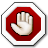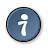Search
»»»Home «««
Framework
Screenshots
FAQ
»»»TELL «««
Types
Operators
Functions
Example
»»»Toped «««
Database
Cells
Select
Edit
Properties
Rendering
GUI
Miscellaneous
»»»Ifaces «««
GDSII
OASIS
CIF
DRC

## Functions

Functions are the basic building blocks of TELL code. Syntactically they look like C code. Below is the list of the main properties of TELL functions

• Functions can be prototyped
• There is no main functions as in C. Instead code is allowed outside function boundaries as described in the introduction
• Functions can be overloaded as long as their type remains fixed.
• Functions accept arguments and return a value. void keyword is predefined to allow a definition of the function that does not return anything.
• Functions can be called recursively.

The example below demonstrate the function definition and function call.

```   box MOStran(point bound, real W, real L) {
// draw some shapes
return {{0,0},{10,8}}; // overlapping box
}

void Invertor(point bound, real NW, real NL, real PW, real PL) {
MOStran(bound,NW,NL);
// doing something
box ptrActive = MOStran(bound,PW,PL);
}```

An example of overloaded functions are Toped build-in functions like addbox, addpoly etc. described later in this manual.

WarningSome overloaded definitions may be ambiguous. If a function is called with anonymous arguments, and more than one of the definitions match the argument list, the result of the call is undefined. Here is an example:``` struct same_as_point {real a; real b}; function ambi (point A) { echo("Input argument of type point); // do something } function ambi (same_as_point A) { echo("Input argument of type same_as_point); // do something } point P = {1,1}; ambi({1,1}); // ambiguous call, any of the overloaded functions // can be called ambi(P); // OK, because P is of a known type```The parser is not flagging this type of errors yet.

### Callbacks

Tell defines a dedicated data type for callback handling. It follows the function declaration syntax with two differences:

• callback keyword marks the start of the declaration.
• the argument list is anonymous
• the function name is either omitted or replaced with the callback type name

Two forms are possible. The second is anonymous.

Callback declarationscallback `return_type` `type_name` ([`argtype` [, `argtype` […]]])callback `return_type` ([`argtype` [, `argtype` […]]]) `var_name``___________________________________________` return_type the return type of the functions which references can be assigned to this type type_name the name of the type which is declared here argtype the type of the function arguments var_name the name of the variable when anonymous callback declaration is used

Although admittedly a bit verbose, callback declaration syntax ensure that all necessary checks can be done in parse time. Once declared, the callback type can be used exactly as any other Tell type.

Only the names of known functions can be assigned to a callback variables. They have to be prefixed by & as in the examples below.

```  int simpleAdd(int a, int b)  {    return (a + b);  }

int simpleSub(int a, int b)  {    return (a - b);  }

// define a callback type unifunctype ...
callback int unifunctype(int,int);

// ... and a variable unifunc of that type

printf("Callback of type unifuncA - returns %d\n",unifuncA(2,3));
unifuncA = &simpleSub;
printf("Callback of type unifuncA - returns %d\n",unifuncA(2,3));

// or define directly a variable unifunc of anonymous callback type
callback int (int,int) unifuncB = &simpleAdd;

printf("Callback of type unifuncB - returns %d\n",unifuncB(2,3));
unifuncB = &simpleSub;
printf("Callback of type unifuncB - returns %d\n",unifuncB(2,3));

//same for the function argument list:
//... using a pre-defined callback type
void funcArguFuncA(int a, int b, unifunctype unifunc)
{
int result = unifunc(a,b);
string buffer = sprintf ("%d fparam %d is %d", a, b, result);
printf ("[%s] \n",buffer);
}

// ... or anonymous callback type
void funcArguFuncB(int a, int b, callback int (int,int) unifunc)
{
int result = unifunc(a,b);
string buffer = sprintf ("%d fparam %d is %d", a, b, result);
printf ("[%s] \n",buffer);
}

funcArguFuncB(2,3,&simpleSub);
funcArguFuncA(2,3,&simpleSub);```

### Recursive function call

Here is a quick example of a function calling itself

```int factoriel(real stop)
{
if (stop > 0)
{
int inter = factoriel(stop - 1);
return (stop * inter);
};
return 1;
}```

top

## Library functions

TELL itself defines predominantly general purpose functions. Toped in turn internally defines and implements a list of functions ensuring its own functionality. In general the editor that interprets TELL shall define and implement its own internal functions. These functions will be parsed first as a part of the initialization phase of the interpreter, so that any internal commands (function calls) received from the command line (or file) can be properly handled.

TELL functions described below are using directly the underlying C functions and more detailed description can be obtained directly from the C math library documentation. One of the few differences is that the trigonometric functions work with angle degrees instead of radians.

### Algebraic functions

function Description
real abs ( real X ) Returns the absolute value of the argument.
int ceil ( real X ) Returns the smallest integer not less than the argument
int floor ( real X ) Returns the largest integer not greater than the argument
int round ( real X ) Returns the nearest integer to the argument.
real sqrt ( real X ) Returns the non-negative square root of the argument
real fmod ( real X, real Y ) Returns the floating-point remainder of dividing X by Y
real pow ( real X, real Y ) Returns the value of X raised to the power of Y.

top

### Trigonometric functions

function Description
real sin ( real X ) Returns the sine of the argument X where X is given in degrees
real cos ( real X ) Returns the cosine of the argument X where X is given in degrees
real tan ( real X ) Returns the tangent of the argument X where X is given in degrees
real asin ( real Y ) Returns the inverse sine; that is the value whose sine is Y. Result is in degrees
real acos ( real Y ) Returns the inverse cosine; that is the value whose cosine is Y. Result is in degrees
real atan ( real Y ) Returns the inverse tangent; that is the value whose tangent is Y. Result is in degrees

Example 1. Trigonometric functions

```   point list arc(point center, int radius, int start, int stop, int numsteps)
{
real step = abs(stop -start) / numsteps;
real current = start;
point list the_arc;
while (current <= stop)
{
point cur_point;
cur_point.x = center.x + radius * cos(current);
cur_point.y = center.y + radius * sin(current);
current = current + step;
the_arc[:+] = cur_point;
}
return the_arc;
}```

top

### Logarithmic functions

function Description
real exp ( real X ) Returns the value of e (the base of natural logarithms) raised to the power of X
real log ( real X ) Returns the natural logarithm of X
real log10 ( real X ) Returns the base 10 logarithm of X.

top

### Hyperbolic functions

function Description
real sinh ( real X ) Return the hyperbolic sine of X
real cosh ( real X ) Return the hyperbolic cosine of X
real tanh ( real X ) Return the hyperbolic tangent of X
real asinh( real Y ) Return the inverse hyperbolic sine; that is the value whose hyperbolic sine is Y.
real acosh( real Y ) Return the inverse hyperbolic cosine; that is the value whose hyperbolic cosine is Y.
real atanh( real Y ) Return the inverse hyperbolic tangent; that is the value whose hyperbolic tangent is Y.

top

### length

Returns the list length. Empty lists are valid and their length will be 0

int length( `llst` )list llst input list

Example 2. length

```   int list b;
echo(length(b));```

top

### echo

Prints the value of a TELL variable. This function is rather temporary. It takes any type of argument and prints its value in the log window. It could be useful for some very basic debugging of the TELL script.

void echo( `variable` )variable A variable of any valid TELL type.

Example 3. echo

```   echo("Welcome");
point list triangle{{0,0},{1,2},{3,4}};
echo (triangle);```

top

### printf

Write formatted data to the Tell log. This function mimics the syntax of the corresponding C function and works in a similar way. It implements the functionality of the C format tags including the flags, width, precision and length parameters.

void printf( `format` [,`param` [,`param` [,…]]] )string format The text to be written on the log console. It can optionally contain format tags. param Zero or more comma separated variables. Their number and types shall strictly correspond to the tags in the format string. Legal types are int, real and string. The function won’t accept any of the Tell specific or user defined types.

The differences with the standard C printf implementation are listed below:

• the function type is void
• the stdout is replaced by the Tell console.
• the format specifiers listed below shall not be used and might produce undefined results:

• c - character
• n - expecting a pointer argument

The definition of the format tags is widely available on external C/C++ reference sources - for example here

top

### sprintf

Write formatted data to a string. Exactly like printf, but instead of the log console it writes all the output in the returned string.

string sprintf( `format` [,`param` [,`param` [,…]]] )string format The text to be written on the log console. It can optionally contain format tags. param Zero or more comma separated variables. Their number and types shall strictly correspond to the tags in the formatting string. Legal types are int, real and string. The function won’t accept any of the Tell specific or user defined types.

The differences with the standard C implementation of sprintf are listed below:

• the function type is string
• the format specifiers listed below shall not be used and might produce undefined results:

• c - character
• n - expecting a pointer argument

The definition of the format tags is widely available on external C/C++ reference sources - for example here

top

### exec

Executes external OS command. Current implementation is rather experimental. The function itself doesn’t return a result or status. The standard input and output is redirected to the Toped console and is highly dependent on the platform.

void exec(`argument`)string argument external command to be executed

The primary purpose of this function is to launch external scripts.

Example 4. exec

```   exec("ls");  //On Linux
exec("dir"); //On Windows```

top

### exit

Exits the current session. Intended to be used from TELL scripts. If database contains unsaved objects the function will still ask to save it before exiting.

void exit()`-`

top